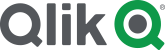#Community

Highlighted
New Contributor II

## Scenario Planning Model - input variable for each variable category combination

Hi,

TLDR:

I needed to do something similar to the person in this post: https://community.qlik.com/t5/Qlik-Sense-App-Development/InputField-and-InputSum-in-QlikSense/td-p/4.  What I am trying to understand is how do you create an input variable for each category (because they require different calculations) without using nested if statements. I dont think nested if statements will work because the combination of input variables will require 50+ nested if statements.

Background:

In order to accomplish this, I created a basic scenario planning table in haste without spending too much time on thinking about how to model it properly. The goal of the model is  to analyze cost savings based on inputs. It is a simple model, you have one variable (Level) and seven categories within Level (1-7). Using the variable extension, seven different sliders are used (because they have different calculations), so that you can adjust each category accordingly. Below is sample code. As you can see,  all the calculations are in the front end, uses nested IF formulas, its really messy and I am sure there is a much cleaner way of doing this. Not to mention, this code is repeated, for each business, this is just for one business.

if([ELT Level]=1 and count({\$<SBU={"US"},[Is Manager]={"No"}>}[Full Name])<=0, (sum({\$<SBU={"US"},[Is Manager]={"No"}>}Median)+(median({1<[Is Manager]={"No"}>}[Median])*vUSnumemp1)),
if([ELT Level]=1,(sum({\$<SBU={"US"},[Is Manager]={"No"}>}Median)+(median({\$<SBU={"US"},[Is Manager]={"No"}>}[Median])*vUSnumemp1)),
if([ELT Level]=2 AND count({\$<SBU={"US"},[Is Manager]={"No"}>}[Full Name])<=0, (sum({\$<SBU={"US"},[Is Manager]={"No"}>}Median)+(median({1<[Is Manager]={"No"}>}[Median])*vUSnumemp2)),
if([ELT Level]=2,(sum({\$<SBU={"US"},[Is Manager]={"No"}>}Median)+(median({\$<SBU={"US"},[Is Manager]={"No"}>}[Median])*vUSnumemp2)),
if([ELT Level]=3 and count({\$<SBU={"US"},[Is Manager]={"No"}>}[Full Name])<=0, (sum({\$<SBU={"US"},[Is Manager]={"No"}>}Median)+(median({1<[Is Manager]={"No"}>}[Median])*vUSnumemp3)),
if([ELT Level]=3,(sum({\$<SBU={"US"},[Is Manager]={"No"}>}Median)+(median({\$<SBU={"US"},[Is Manager]={"No"}>}[Median])*vUSnumemp3)),
if([ELT Level]=4 and count({\$<SBU={"US"},[Is Manager]={"No"}>}[Full Name])<=0, (sum({\$<SBU={"US"},[Is Manager]={"No"}>}Median)+(median({1<[Is Manager]={"No"}>}[Median])*vUSnumemp4)),
if([ELT Level]=4,(sum({\$<SBU={"US"},[Is Manager]={"No"}>}Median)+(median({\$<SBU={"US"},[Is Manager]={"No"}>}[Median])*vUSnumemp4)),
if([ELT Level]=5 and count({\$<SBU={"US"},[Is Manager]={"No"}>}[Full Name])<=0, (sum({\$<SBU={"US"},[Is Manager]={"No"}>}Median)+(median({1<[Is Manager]={"No"}>}[Median])*vUSnumemp5)),
if([ELT Level]=5,(sum({\$<SBU={"US"},[Is Manager]={"No"}>}Median)+(median({\$<SBU={"US"},[Is Manager]={"No"}>}[Median])*vUSnumemp5)),
if([ELT Level]=6 and count({\$<SBU={"US"},[Is Manager]={"No"}>}[Full Name])<=0, (sum({\$<SBU={"US"},[Is Manager]={"No"}>}Median)+(median({1<[Is Manager]={"No"}>}[Median])*vUSnumemp6)),
if([ELT Level]=6,(sum({\$<SBU={"US"},[Is Manager]={"No"}>}Median)+(median({\$<SBU={"US"},[Is Manager]={"No"}>}[Median])*vUSnumemp6)),
if([ELT Level]=7 and count({\$<SBU={"US"},[Is Manager]={"No"}>}[Full Name])<=0, (sum({\$<SBU={"US"},[Is Manager]={"No"}>}Median)+(median({1<[Is Manager]={"No"}>}[Median])*vUSnumemp7)),
if([ELT Level]=7,(sum({\$<SBU={"US"},[Is Manager]={"No"}>}Median)+(median({\$<SBU={"US"},[Is Manager]={"No"}>}[Median])*vUSnumemp7)),
if([ELT Level]=8 and count({\$<SBU={"US"},[Is Manager]={"No"}>}[Full Name])<=0, (sum({\$<SBU={"US"},[Is Manager]={"No"}>}Median)+(median({1<[Is Manager]={"No"}>}[Median])*vUSnumemp8)),
if([ELT Level]=8,(sum({\$<SBU={"US"},[Is Manager]={"No"}>}Median)+(median({\$<SBU={"US"},[Is Manager]={"No"}>}[Median])*vUSnumemp8)),
0
))))))))))))))))

Now I need to make the model more complex by adding another variable (city) that has 15 categories, but the functionality still needs to remain the same: adjust the input by level AND city  which if I use this same approach, would require like ~50+ nested if formulas. I suspect this will decrease performance, but also, simply bothers me as it is ugly code.

So my question is, how do I do this in a more concise, clean manner. One thought I had was creating another table,  which lists the cost of every combination (i.e., level by city), and than applying the variable to that table. However, I am still unsure on how to get around using the nested if statements so that each input is correctly applied to the right combination, which is really what I am trying to understand.

Edit: I tried using set analysis, but it did not work, the only thing that seems to work is using an If statement to add the slider variables to each level as shown above.

Thanks!

Labels (3)